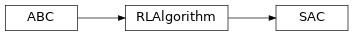# garage.torch.algos.sac¶

This modules creates a sac model in PyTorch.

class SAC(env_spec, policy, qf1, qf2, replay_buffer, *, max_episode_length_eval=None, gradient_steps_per_itr, fixed_alpha=None, target_entropy=None, initial_log_entropy=0.0, discount=0.99, buffer_batch_size=64, min_buffer_size=int(10000.0), target_update_tau=0.005, policy_lr=0.0003, qf_lr=0.0003, reward_scale=1.0, optimizer=torch.optim.Adam, steps_per_epoch=1, num_evaluation_episodes=10, eval_env=None, use_deterministic_evaluation=True)A SAC Model in Torch.

Based on Soft Actor-Critic and Applications:

https://arxiv.org/abs/1812.05905

Soft Actor-Critic (SAC) is an algorithm which optimizes a stochastic policy in an off-policy way, forming a bridge between stochastic policy optimization and DDPG-style approaches. A central feature of SAC is entropy regularization. The policy is trained to maximize a trade-off between expected return and entropy, a measure of randomness in the policy. This has a close connection to the exploration-exploitation trade-off: increasing entropy results in more exploration, which can accelerate learning later on. It can also prevent the policy from prematurely converging to a bad local optimum.

Parameters
• policy (garage.torch.policy.Policy) – Policy/Actor/Agent that is being optimized by SAC.

• qf1 (garage.torch.q_function.ContinuousMLPQFunction) – QFunction/Critic used for actor/policy optimization. See Soft Actor-Critic and Applications.

• qf2 (garage.torch.q_function.ContinuousMLPQFunction) – QFunction/Critic used for actor/policy optimization. See Soft Actor-Critic and Applications.

• replay_buffer (ReplayBuffer) – Stores transitions that are previously collected by the sampler.

• env_spec (EnvSpec) – The env_spec attribute of the environment that the agent is being trained in.

• max_episode_length_eval (int or None) – Maximum length of episodes used for off-policy evaluation. If None, defaults to env_spec.max_episode_length.

• gradient_steps_per_itr (int) – Number of optimization steps that should

• gradient_steps_per_itr – Number of optimization steps that should occur before the training step is over and a new batch of transitions is collected by the sampler.

• fixed_alpha (float) – The entropy/temperature to be used if temperature is not supposed to be learned.

• target_entropy (float) – target entropy to be used during entropy/temperature optimization. If None, the default heuristic from Soft Actor-Critic Algorithms and Applications is used.

• initial_log_entropy (float) – initial entropy/temperature coefficient to be used if a fixed_alpha is not being used (fixed_alpha=None), and the entropy/temperature coefficient is being learned.

• discount (float) – Discount factor to be used during sampling and critic/q_function optimization.

• buffer_batch_size (int) – The number of transitions sampled from the replay buffer that are used during a single optimization step.

• min_buffer_size (int) – The minimum number of transitions that need to be in the replay buffer before training can begin.

• target_update_tau (float) – coefficient that controls the rate at which the target q_functions update over optimization iterations.

• policy_lr (float) – learning rate for policy optimizers.

• qf_lr (float) – learning rate for q_function optimizers.

• reward_scale (float) – reward scale. Changing this hyperparameter changes the effect that the reward from a transition will have during optimization.

• optimizer (torch.optim.Optimizer) – optimizer to be used for policy/actor, q_functions/critics, and temperature/entropy optimizations.

• steps_per_epoch (int) – Number of train_once calls per epoch.

• num_evaluation_episodes (int) – The number of evaluation episodes used for computing eval stats at the end of every epoch.

• eval_env (Environment) – environment used for collecting evaluation episodes. If None, a copy of the train env is used.

• use_deterministic_evaluation (bool) – True if the trained policy should be evaluated deterministically.

train(self, trainer)

Obtain samplers and start actual training for each epoch.

Parameters

trainer (Trainer) – Gives the algorithm the access to :method:~Trainer.step_epochs(), which provides services such as snapshotting and sampler control.

Returns

The average return in last epoch cycle.

Return type

float

train_once(self, itr=None, paths=None)

Complete 1 training iteration of SAC.

Parameters
• itr (int) – Iteration number. This argument is deprecated.

• paths (list[dict]) – A list of collected paths. This argument is deprecated.

Returns

loss from actor/policy network after optimization. torch.Tensor: loss from 1st q-function after optimization. torch.Tensor: loss from 2nd q-function after optimization.

Return type

torch.Tensor

optimize_policy(self, samples_data)

Optimize the policy q_functions, and temperature coefficient.

Parameters

samples_data (dict) – Transitions(S,A,R,S’) that are sampled from the replay buffer. It should have the keys ‘observation’, ‘action’, ‘reward’, ‘terminal’, and ‘next_observations’.

Note

samples_data’s entries should be torch.Tensor’s with the following shapes:

observation: $$(N, O^*)$$ action: $$(N, A^*)$$ reward: $$(N, 1)$$ terminal: $$(N, 1)$$ next_observation: $$(N, O^*)$$

Returns

loss from actor/policy network after optimization. torch.Tensor: loss from 1st q-function after optimization. torch.Tensor: loss from 2nd q-function after optimization.

Return type

torch.Tensor

property networks(self)

Return all the networks within the model.

Returns

A list of networks.

Return type

list

to(self, device=None)

Put all the networks within the model on device.

Parameters

device (str) – ID of GPU or CPU.